# NEET Chemistry Reaction Mechanism - Organic Chemistry Questions Solved

Reactivity order of halides for dehydrohalogenation is

(a) R-F>R-Cl>R-Br>R-I(b) R-I>R-Br>R-Cl>R-F(c) R-I>R-Cl>R-Br>R-F(d) R-F>R-I>R-Br>R-Cl

Complete Question Bank + Test Series
Complete Question Bank

Difficulty Level:

The increasing order of hydrolysis of the following compounds: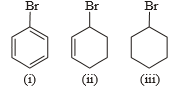(a) (i)<(iii)<(ii)

(b) (ii)<(iii)<(i)

(c) (ii)<(i)<(iii)

(d) (i)<(ii)<(iii)

Complete Question Bank + Test Series
Complete Question Bank

Difficulty Level:

What is major product of following reaction ?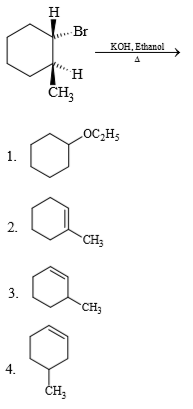Complete Question Bank + Test Series
Complete Question Bank

Difficulty Level: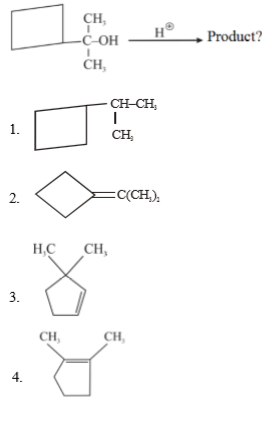Complete Question Bank + Test Series
Complete Question Bank

Difficulty Level:

Consider the given reaction below: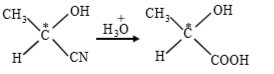Which of these is true regarding the reaction shown above?

(1) the configuration of the chiral carbon remains same.

(2) the configuration of the chiral carbon gets inverted

(3) the  compound formed as a product must be a dextro isomer

(4) the reactant is optically inactive but the product is obtained as a levo isomer.

Complete Question Bank + Test Series
Complete Question Bank

Difficulty Level: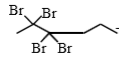$\underset{∆}{\overset{\mathrm{Zn}}{\to }}$ A$\underset{{\mathrm{NH}}_{3}/\mathrm{EtOH}}{\overset{\mathrm{Na}}{\to }}$ B

Product 2 is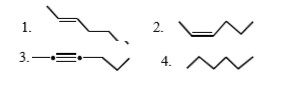Complete Question Bank + Test Series
Complete Question Bank

Difficulty Level:

Complete Question Bank + Test Series
Complete Question Bank

Difficulty Level:

Which of the following is formed by the thermal decomposition of the hydroxide of: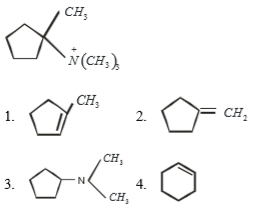Complete Question Bank + Test Series
Complete Question Bank

Difficulty Level: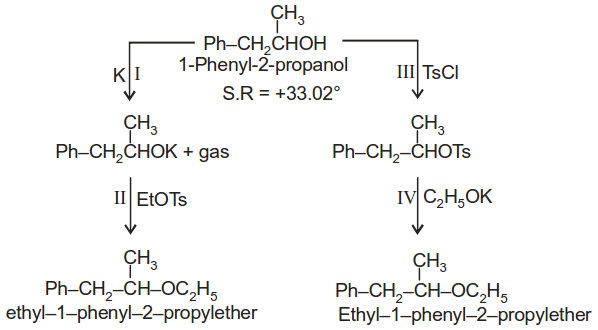(1) Both the ethers obtained by the two routes have opposite but equal optical rotation.

(2) One of the ether is obtained as a racemic mixture.

(3) Step II & III both are SN2 reaction and both have inversion.

(4) Step II has inversion but step III has retention.

Complete Question Bank + Test Series
Complete Question Bank

Difficulty Level:

P is

(1) Ph–O–SO2OMe

(2) PhOMe

(3) PhOSO2OPh

(4) PhMe